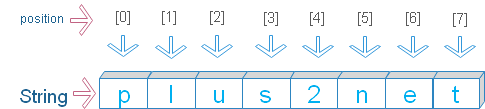# Palindrome in Python

A string is said Palindrome if it is same when reversed.
Examples of Palindrome : level, racecar , radar , malayalam

## Checking By using reversed()

We can reverse the sequence of all the elements of any iterable object by using using reversed() function . String method join() creates a string by joining all the elements.
``````str='level'
str_rev=''.join(reversed(str))
if(str==str_rev):
print("The string ",str," is a Palindrome ")
else:
print("The string ",str," is NOT a Palindrome ") ``````
Output
``The string  level  is a Palindrome``

## By looping & length

By using for loop we can reverse a sting. The function len() will return the length of the string. Using the length of the string we can start from the last position char and come upto the first char.
``````str='plus2net'
n=len(str) # length of the string
x=''
for i in range(n-1,-1,-1):
#print(str[i],end='')
x=x+str[i]
if(x==str):
print(" \n The string ",str," is a Palindrome ")
else:
print("\n The string ",str," is NOT a Palindrome ")``````
Output
``The string  plus2net  is NOT a Palindrome``

## By using string sliceThis is the shortest code to reverse a string.
``````str=input("Enter a string: ")
str_rev=str[::-1]
if(str_rev==str):
print(" \n The string ",str," is a Palindrome ")
else:
print("\n The string ",str," is NOT a Palindrome ")``````
Output
``````Enter a string: radar

The string  radar  is a Palindrome``````

## for loop

All strings are iterable object so we can use for loop to iterate through the string.
``````str=input("Enter any string : ")
x=''
for i in str:
x=i+x
if(x==str):
print(" \n The string ",str," is a Palindrome ")
else:
print("\n The string ",str," is NOT a Palindrome ")``````
Output
``````Enter any string : malayalam

The string  malayalam  is a Palindrome ``````

## Case insensitive

All above codes are case sensitive. By making the string to upper case ( upper() ) or by making to lower case ( lower() ) we can check for Palindrome without case sensitive.
``````str=input("Enter a string: ")
str1=str.upper()
str_rev=str1[::-1]
if(str_rev==str1):
print(" \n The string ",str," is a Palindrome ")
else:
print("\n The string ",str," is NOT a Palindrome ")``````
Output
``````Enter a string: Radar

The string  Radar  is a Palindrome``````

Subscribe to our YouTube Channel here

## Subscribe

* indicates required
Subscribe to plus2netplus2net.com